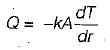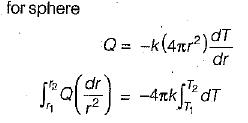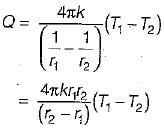Courses

# Test: One-Dimensional Steady-state Conduction - 1

## 10 Questions MCQ Test Topicwise Question Bank for Mechanical Engineering | Test: One-Dimensional Steady-state Conduction - 1

Description
This mock test of Test: One-Dimensional Steady-state Conduction - 1 for Chemical Engineering helps you for every Chemical Engineering entrance exam. This contains 10 Multiple Choice Questions for Chemical Engineering Test: One-Dimensional Steady-state Conduction - 1 (mcq) to study with solutions a complete question bank. The solved questions answers in this Test: One-Dimensional Steady-state Conduction - 1 quiz give you a good mix of easy questions and tough questions. Chemical Engineering students definitely take this Test: One-Dimensional Steady-state Conduction - 1 exercise for a better result in the exam. You can find other Test: One-Dimensional Steady-state Conduction - 1 extra questions, long questions & short questions for Chemical Engineering on EduRev as well by searching above.
QUESTION: 1

Solution:
QUESTION: 2

### Consider the following statements: The Fourier heat conduction equation presumes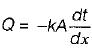1. Steady state conditions 2. Constant value of thermal conductivity 3. Uniform temperature at the wait surfaces 4. One - dimensional heat flow. Which of these statements are correct?

Solution:

k may be constant or vary.

QUESTION: 3

### The heat conducted through a wall of thickness δ is given by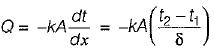Which amongst the following is not valid pertaining to above equation?

Solution:
QUESTION: 4

One end of iron rod of cross-sectional area A and length L is kept in a steambath. After same time steady state conditions prevail and Q unit of heat per second passes through any cross- section of the rod. The heat flow rate will be adversely affected if

Solution: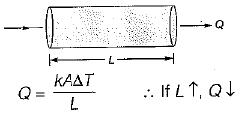QUESTION: 5

Match the List-1 (Coordinate system) with ListII (Steady state governing equation) the following: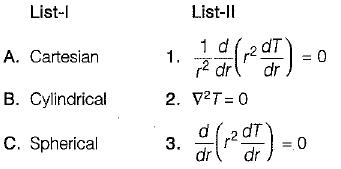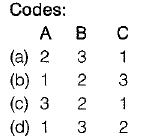Solution:
QUESTION: 6

Temperature distribution in a cylindrical rod with heat generation is given by

Solution:
QUESTION: 7

The Fourier law of heat conduction is valid for

Solution:

While deriving Fourier law of heat conduction some assumptions are made, which are
1. Steady-state condition
2. One-directional heat transfer
3. No heat generation
4. Material is homogeneous and isotropic

QUESTION: 8

Fourier law of heat conduction is given by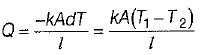where
I = thickness of the slab
dT = temperature difference
In this term l/kA is given by

Solution:

In case of plane slab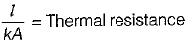QUESTION: 9

Heat transfer by conduction through hollow cylinder is given by

Solution:

From Fourier law of heat conduction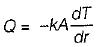For hollow cylinder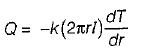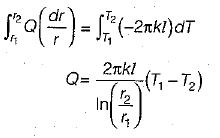QUESTION: 10

Heat transfer by conduction through sphere is given by

Solution:

From Fourier law of heat conduction